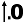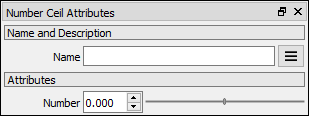# Number Ceil node

Left Toolbar >Math Nodes >Number Ceil

The Number Ceil node returns the smallest integer greater than or equal of the Number value.

## Procedure

### To calculate the ceil of the number in the node graph:

1. On the left toolbar choose Math Nodes> Number Ceil2. Drag the Number Ceil icon into the Node Graph.3. Connect the Output: result.

## Inputs

number A NUMBER type of value.

## Outputs

Result The number value.

## Attributes PanelNumber Number value. This attribute is exposed as input by default.

Math Nodes

Main Page